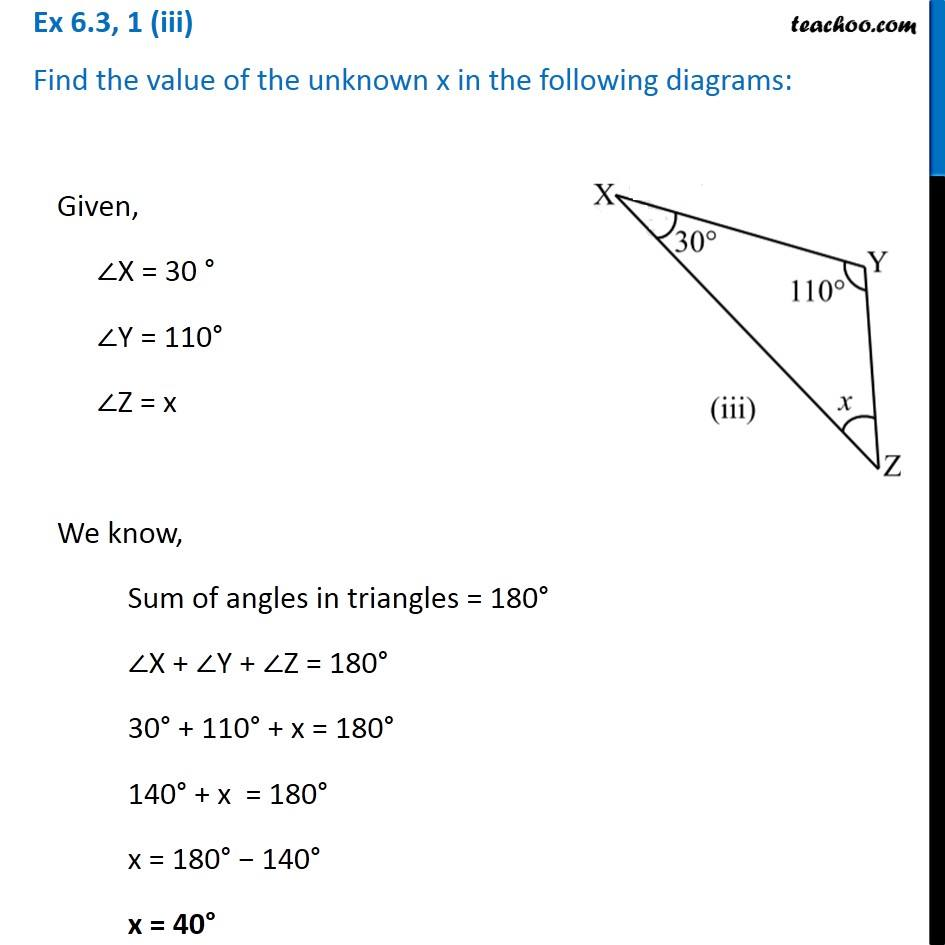Ex 6.3

Chapter 6 Class 7 Triangle and its Properties
Serial order wiseLearn in your speed, with individual attention - Teachoo Maths 1-on-1 Class

### Transcript

Ex 6.3, 1 (iii) Find the value of the unknown x in the following diagrams: Given, ∠X = 30 ° ∠Y = 110° ∠Z = x We know, Sum of angles in triangles = 180° ∠X + ∠Y + ∠Z = 180° 30° + 110° + x = 180° 140° + x = 180° x = 180° − 140° x = 40°